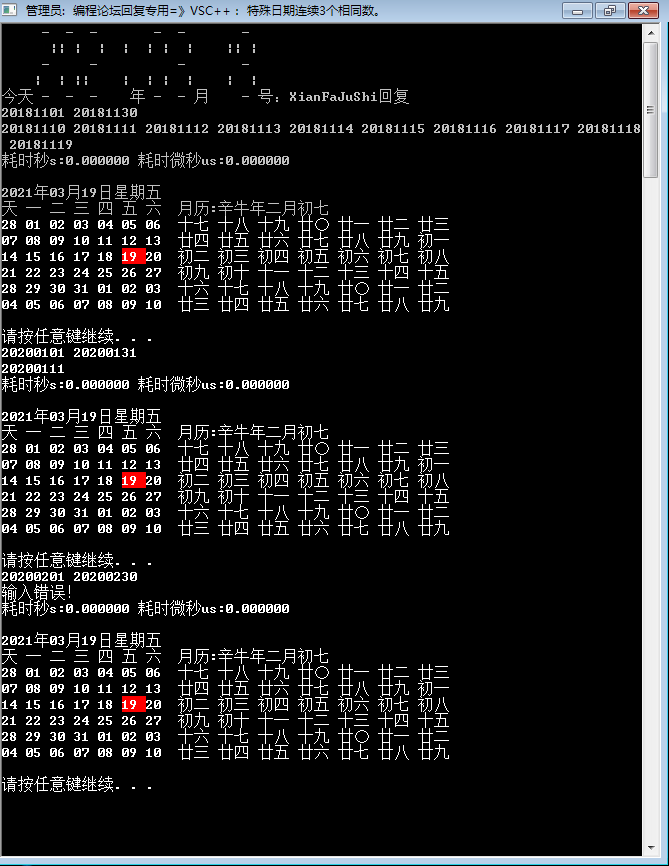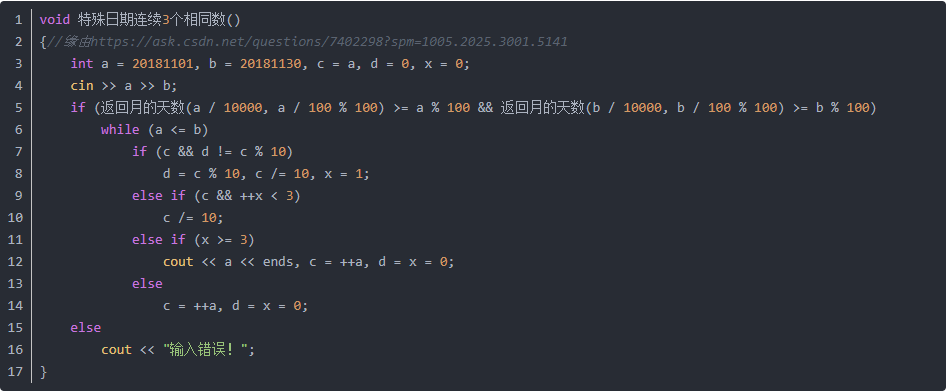2021-02-25 18:43

# 第二届蓝桥杯试题B：特殊日期

``````#include<stdio.h>
int trial(int num)/*判断是否为合法日期*/
{int mon,day,year,t=1;
year=num/10000;
mon=num%10000;
if(mon<1000) return 0;
day=mon%100;
mon=mon/100;
if(day==0||day>=32||mon<=0||mon>=13) return 0;
switch(mon)
{case 4:case 6:case 9:case 11:if(day>30)t=0;break;
case 2:if(year%4==0&&year%100!=0||year%400==0)
{if(day>29)t=0;}
else if(day>28)t=0;}
return t;}
int main()
{int a,b;
int num=0,i,t=1,j;
scanf("%d%d",&a,&b);/*输入两个8位数日期，例如20181101 20181130*/
for(i=a;i<=b;i++)
{for(j=10;j<=1e7;j*=10)
if(i/j%10==i/(j/10)%10)
{t++;if(t==3)break;}/*问题所在，不知道为什么没算20181111在内*/
else t=1;
if(t==3&&trial(i)==1)num++;}/*满足出现3个连续数及属于合法日期的数会被记录*/
printf("%d\n",num);/*输出符合条件的数的个数*/
return 0;}``````

• 点赞
• 写回答
• 关注问题
• 收藏
• 复制链接分享
• 邀请回答

#### 1条回答

•点赞 1 评论 复制链接分享